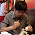2016年11月17日 星期四

機器學習(1)--使用OPENCV KNN實作手寫辨識

這一篇我們要來利用OPENCV 所提供的kNN(k-Nearest Neighbour ）來實作手寫辨識。在程式實作中，我稍微改變了OPENCV官版原本的範例程式，除了修正在Python3.5+OPENCV3.x
build code 會error以外，程式最後並加入自己手畫的數字圖進行預測。可以使用小畫家直接手繪一20x20 pixel 黑底白字數字圖當作自己輸入預測的樣本。如下<圖一>

<圖一>上方為輸入的手寫樣本圖片，下方是使用OPENCV KNN辨識出的結果

kNN(k-Nearest Neighbour ）基本原理
kNN 可以說是最簡單的監督學習分類器了。想法也很簡單，就是找出測試 資料在特徵空間中的最近鄰居。我們將使用下面的圖片介紹它。

上圖中的物件可以分成兩組，藍色方塊和紅色三角。每一組也可以稱為一 個類。我們可以把所有的這些物件看成是一個城鎮中房子，而所有的房子分別屬於藍色和紅色家族，而這個城鎮就是所謂的特徵空間。
現在城鎮中來了一個新人，他的新房子用綠色圓盤表示。我們要根據他房子的位置把他歸為藍色家族或紅色家族。我們把這過程成為分類。我們應該怎麼做呢？
一個方法就是查看他最近的鄰居屬於那個家族，從圖像中我們知道最近的 是紅色三角家族。所以他被分到紅色家族。這種方法被稱為簡單近鄰，因為分 類僅僅決定與它最近的鄰居。但是這裡還有一個問題。紅色三角可能是最近的，但如果他周圍還有很多 藍色方塊怎麼辦呢？此時藍色方塊對局部的影響應該大於紅色三角。所以僅僅檢測最近的一個鄰居是不足的。所以我們檢測k 個最近鄰居。誰在這 k 個鄰 居中佔據多數，那新的成員就屬於誰那一類。如果  k  等於 3，也就是在上面圖像中檢測 3 個最近的鄰居。他有兩個紅的和一個藍的鄰居，所以他還是屬於紅色家族。但是如果 k 等於 7 呢？他有 5 個藍色和 2 個紅色鄰居，現在他就會 被分到藍色家族了。
k 的取值對結果影響非常大。更有趣的是，如果 k 等於 4 呢？兩個紅兩個藍。這是一個死結。所以 k 的取值最好為奇數。這中根據 k 個最近鄰居進行分類的方法被稱為kNN。

由於OPENCV3.0之後對於ML函式庫的支援不完整，原因是有些機器學習的演算法有專利版權並非全部面費開放，包含之後所會用到的SVM演算法也是，所以要另外再安裝OPENCV 的contrib，這是可以給教育免費所使用的套件。也因此OPENCV3.x之後所引用ML的演算法跟之前OPENCV2.x的版本有些不同。
對於使用windows環境的可以直接從下列網址：下載已build 好的OPENCV_contrib直接用
pip install安裝。

http://www.lfd.uci.edu/~gohlke/pythonlibs/#scipy

opencv_python-3.1.0+contrib_opencl-cp35-cp35m-win32.whl

https://github.com/opencv/opencv_contrib

接下來就來實作這個範例程式，OPENCV source 裡有附一張1000x2000 pixel的手寫數字圖片，我們把它切成一小塊20x20 pixel的小圖就代表著每一個手寫數字。切割完後可以得到5000筆這樣的小圖當訓練及測試樣本，各占一半。作法會先把圖片灰值化後再把像素展平成一列20x20=400像素，這些2500張訓練樣本的400個的灰值像素即當成特徵值進行KNN訓練，之後再利用另一半測試樣本進行預測，可以得出準確率91.7% ，如下圖。
但是在估算完準確率之後，我再進行自己用小畫家產生手寫的圖片進行辨識，結果如下

<圖一>上方為輸入的手寫樣本圖片，下方是使用OPENCV KNN辨識出的結果

<完整範例程式>

import numpy as np
import cv2
from matplotlib import pyplot as plt

#1.載入原始圖像並灰值化，原始影像為1000x2000 ，3 channel
print("img shape=",img.shape)
gray = cv2.cvtColor(img,cv2.COLOR_BGR2GRAY)
print("gray shape=",gray.shape)

#2.切成每一小塊20x20 pixel
#先將gray 1000x2000 [rowsxcols] pixel , 將row=1000/50 ：意為50列20pixel單位
#再將產生的cols =2000/100 =>意為100行20pixel單位
cells = [np.hsplit(row,100) for row in np.vsplit(gray,50)]

#3.將cells為一50x100 的list轉成array (50,100,20,20)
x = np.array(cells)
print("x shape=",x.shape)

#4.把20x20 pixel 展平成一行400 pixel
#將cells array X 轉成5000x400 後並分成兩半 train's data and test's data
train = x[:,:50].reshape(-1,400).astype(np.float32) # Size = (2500,400)
test = x[:,50:100].reshape(-1,400).astype(np.float32) # Size = (2500,400)
print("train shape=",train.shape)
print("test shape=",test.shape)

#5.Create labels for train and test data
k = np.arange(10)
train_labels = np.repeat(k,250)[:,np.newaxis]
test_labels = train_labels.copy()
print ("train_labels.shape=",train_labels.shape)
print ("test_labels.shape=",test_labels.shape)

#6. Initiate kNN, train the data, then test it with test data for k=1
knn = cv2.ml.KNearest_create()
knn.train(train,cv2.ml.ROW_SAMPLE,train_labels)
ret, result, neighbours, dist = knn.findNearest(test, k=1)

#7. Now we check the accuracy of classification
# For that, compare the result with test_labels and check which are wrong
matches = result==test_labels
correct = np.count_nonzero(matches)
accuracy = correct*100.0/result.size
print (accuracy)

#8.save the data
np.savez('knn_data.npz',train=train, train_labels=train_labels)

##-------------------------------------------------------------------------
##=========================================================================
##======Predict testing====================================================
#A.Now re-load the data
with np.load('knn_data.npz') as data:
print (data.files)
train = data['train']
train_labels = data['train_labels']

#B.輸入自己手寫的image data 必須是20x20 pixel
Input_Numer=*10
img_num =*10
img_res =*10
testData_r=*10
result=*10
result_str=*10
Input_Numer="0.jpg"
Input_Numer="1.jpg"
Input_Numer="2.jpg"
Input_Numer="3.jpg"
Input_Numer="4.jpg"
Input_Numer="5.jpg"
Input_Numer="6.jpg"
Input_Numer="7.jpg"
Input_Numer="8.jpg"
Input_Numer="9.jpg"
font = cv2.FONT_HERSHEY_SIMPLEX

#C.Predicting
for i in range(10):  #input 10 number
testData_r[i]  = img_num[i][:,:].reshape(-1,400).astype(np.float32) # Size = (1,400)
ret, result[i], neighbours, dist = knn.findNearest(testData_r[i], k=5)
#產生white screen以顯示預測結果的白底
img_res[i] = np.zeros((64,64,3), np.uint8)
img_res[i][:,:]=[255,255,255]
#將結果轉成字串以便顯示在圖上
print("result[i] =",result[i].astype(np.int32)) #change type from float32 to int32
result_str[i]=str(result[i].astype(np.int32))
if result[i].astype(np.int32)==i:
cv2.putText(img_res[i],result_str[i],(15,52), font, 2,(0,255,0),3,cv2.LINE_AA)
else:
cv2.putText(img_res[i],result_str[i],(15,52), font, 2,(255,0,0),3,cv2.LINE_AA)
#===顯示輸入與預測結果圖======
Input_Numer_name = ['Input 0', 'Input 1','Input 2', 'Input 3','Input 4',\
'Input 5','Input 6', 'Input 7','Input8', 'Input9']
predict_Numer_name =['predict 0', 'predict 1','predict 2', 'predict 3','predict 4', \
'predict 5','predict6 ', 'predict 7','predict 8', 'predict 9']
for i in range(10):
plt.subplot(2,10,i+1),plt.imshow(img_num[i],cmap = 'gray')
plt.title(Input_Numer_name[i]), plt.xticks([]), plt.yticks([])
plt.subplot(2,10,i+11),plt.imshow(img_res[i],cmap = 'gray')
plt.title(predict_Numer_name[i]), plt.xticks([]), plt.yticks([])
plt.show()

<其他有關OPENCV 文章>
OPENCV(10)--Canny Edge Detection(Canny邊緣檢測)
OPENCV(8)--Histogram & Histograms Equalization(長條圖與長條圖均衡化)
OPENCV(7)--2D Convolution ，Image Filtering and Blurring (旋積，濾波與模糊)
OPENCV(6)--Trackbar(軌道桿)
OPENCV(5)--Drawing
OPENCV(4)--Grayscale,Binarization,Threshole(灰階化,二值化,閥值)
OPENCV(3)--Matplotlib pyplot bassic function
OPENCV(2)--Capture Video from Camera
OPENCV(1 )--How to install OPENCV in Python

<參考資料:>OPENCV官網
http://docs.opencv.org/3.1.0/index.html

5 則留言:

1.太感謝你的分享了!!讓我有了入門的知識

2.想請問一下您那個1000X2000的圖片是怎麼來的??謝謝您

1.作者已經移除這則留言。

3.作者已經移除這則留言。

4.大大您好，想詢問一下當我訓練好Xml檔分類器，如何使用Python來做影像辨識